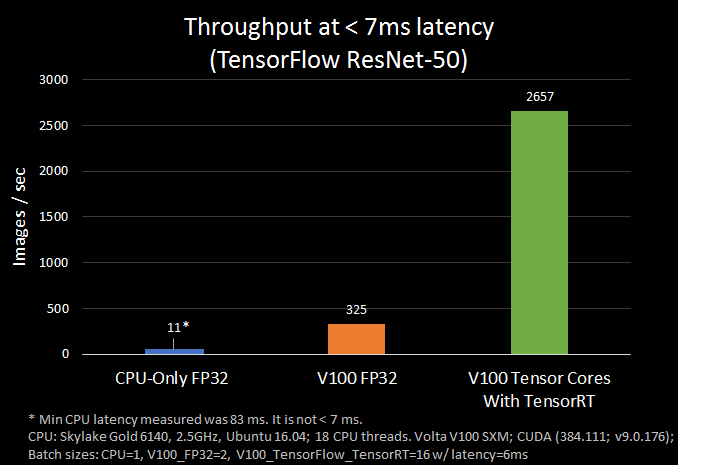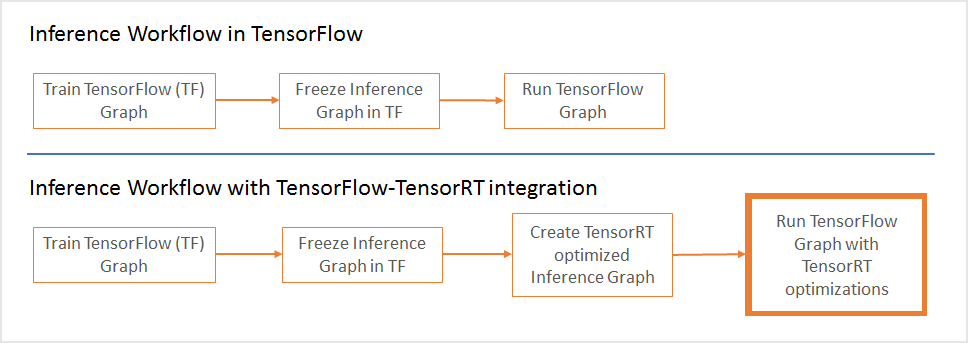# Announcing TensorRT integration with TensorFlow 1.7

March 27, 2018

Posted by Laurence Moroney (Google) and Siddarth Sharma (NVIDIA)

Today we are announcing integration of NVIDIA® TensorRTTM and TensorFlow. TensorRT is a library that optimizes deep learning models for inference and creates a runtime for deployment on GPUs in production environments. It brings a number of FP16 and INT8 optimizations to TensorFlow and automatically selects platform specific kernels to maximize throughput and minimizes latency during inference on GPUs. We are excited about the new integrated workflow as it simplifies the path to use TensorRT from within TensorFlow with world-class performance. In our tests, we found that ResNet-50 performed 8x faster under 7 ms latency with the TensorFlow-TensorRT integration using NVIDIA Volta Tensor Cores as compared with running TensorFlow only.## Sub-Graph Optimizations within TensorFlow

Now in TensorFlow 1.7, TensorRT optimizes compatible sub-graphs and let's TensorFlow execute the rest. This approach makes it possible to rapidly develop models with the extensive TensorFlow feature set while getting powerful optimizations with TensorRT when performing inference. If you were already using TensorRT with TensorFlow models, you know that certain unsupported TensorFlow layers had to be imported manually, which in some cases could be time consuming.

From a workflow perspective, you need to ask TensorRT to optimize TensorFlow's sub-graphs and replace each subgraph with a TensorRT optimized node. The output of this step is a frozen graph that can then be used in TensorFlow as before.During inference, TensorFlow executes the graph for all supported areas, and calls TensorRT to execute TensorRT optimized nodes. As an example, if your graph has 3 segments, A, B and C. Segment B is optimized by TensorRT and replaced by a single node. During inference, TensorFlow executes A, then calls TensorRT to execute B, and then TensorFlow executes C.

The newly added TensorFlow API to optimize TensorRT takes the frozen TensorFlow graph, applies optimizations to sub-graphs and sends back to TensorFlow a TensorRT inference graph with optimizations applied. See the code below as an example.

```# Reserve memory for TensorRT inference engine
gpu_options = tf.GPUOptions(per_process_gpu_memory_fraction = number_between_0_and_1)
...
trt_graph = trt.create_inference_graph(
input_graph_def = frozen_graph_def,
outputs = output_node_name,
max_batch_size=batch_size,
max_workspace_size_bytes=workspace_size,
precision_mode=precision)  # Get optimized graph```

The `per_process_gpu_memory_fraction` parameter defines the fraction of GPU memory that TensorFlow is allowed to use, the remaining being available for TensorRT. This parameter should be set the first time the TensorFlow-TensorRT process is started. As an example, a value of 0.67 would allocate 67% of GPU memory for TensorFlow and the remaining 33 % for TensorRT engines.

The `create_inference_graph` function takes a frozen TensorFlow graph and returns an optimized graph with TensorRT nodes. Let's look at the function's parameters:

• `input_graph_def: `frozen TensorFlow graph
• `outputs:` list of strings with names of output nodes e.g. `["resnet_v1_50/predictions/Reshape_1"]`
• `max_batch_size:` integer, size of input batch e.g. 16
• `max_workspace_size_bytes: `integer, maximum GPU memory size available for TensorRT
• `precision_mode`: string, allowed values "FP32", "FP16" or "INT8"

As an example, if the GPU has 12GB memory, in order to allocate ~4GB for TensorRT engines, set the `per_process_gpu_memory_fraction` parameter to ( 12 - 4 ) / 12 = 0.67 and the max_workspace_size_bytes parameter to 4000000000.

Lets apply the new API to ResNet-50 and see what the optimized model looks like in TensorBoard. The complete code to run the example is available here. The image on the left is ResNet-50 without TensorRT optimizations and the right image is after. In this case, most of the graph gets optimized by TensorRT and replaced by a single node (highlighted).

## Optimized INT8 Inference performance

TensorRT provides capabilities to take models trained in single (FP32) and half (FP16) precision and convert them for deployment with INT8 quantizations at reduced precision with minimal accuracy loss. INT8 models compute faster and place lower requirements on bandwidth but present a challenge in representing weights and activations of neural networks because of the reduced dynamic range available.

 Dynamic Range Minimum Positive Value FP32 -3.4×1038 ~ +3.4×1038 1.4 × 10−45 FP16 65504 ~ +65504 5.96 x 10-8 INT8 -128 ~ +127 1

To address this, TensorRT uses a calibration process that minimizes the information loss when approximating the FP32 network with a limited 8-bit integer representation. With the new integration, after optimizing the TensorFlow graph with TensorRT, you can pass the graph to TensorRT for calibration as below.

`trt_graph=trt.calib_graph_to_infer_graph(calibGraph)`

The rest of the inference workflow remains unchanged from above. The output of this step is a frozen graph that is executed by TensorFlow as described earlier.

## Automatically use Tensor Cores on NVIDIA Volta GPUs

TensorRT runs half precision TensorFlow models on Tensor Cores in VOLTA GPUs for inference. Tensor Cores, provide 8x more throughput than single precision math pipelines. Half precision (also known as FP16) data compared to higher precision FP32 vs FP64 reduces memory usage of the neural network. This allows training and deployment of larger networks, and FP16 data transfers take less time than FP32 or FP64 transfers.

Each Tensor Core performs D = A x B + C, where A, B, C and D are matrices. A and B are half-precision 4x4 matrices, whereas D and C can be either half or single precision 4x4 matrices. The peak performance of Tensor Cores on the V100 is about an order of magnitude (10x) faster than double precision (FP64) and about 4 times faster than single precision (FP32).

## Availability

We are excited about this release and will continue to work closely with NVIDIA to enhance this integration. We expect the new solutions ensure the highest performance possible while maintaining the ease and flexibility of TensorFlow. And as TensorRT supports more networks, you will automatically benefit from the updates without any changes to your code.

To get the new solution, you can use the standard pip install process once TensorFlow 1.7 is released:

`pip install tensorflow-gpu r1.7`

Till then, find detailed installation instructions here: https://github.com/tensorflow/tensorflow/tree/r1.7/tensorflow/contrib/tensorrt

Try it out and let us know what you think!